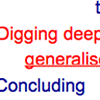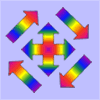# Resources tagged with: Proof by exhaustion

There are 5 NRICH Mathematical resources connected to Proof by exhaustion, you may find related items under Thinking Mathematically.

Broad Topics > Thinking Mathematically > Proof by exhaustion### School Fair Necklaces

##### Age 5 to 11Challenge Level

How many possible necklaces can you find? And how do you know you've found them all?### Mastering Mathematics: the Challenge of Generalising and Proof

##### Age 5 to 11

This article for primary teachers discusses how we can help learners generalise and prove, using NRICH tasks as examples.### Proving

##### Age 7 to 11

These tasks provide opportunities for learners to get better at proving, whether through proof by exhaustion, proof by logical argument, proof by counter example or generic proof.### Code to Zero

##### Age 16 to 18Challenge Level

Find all 3 digit numbers such that by adding the first digit, the square of the second and the cube of the third you get the original number, for example 1 + 3^2 + 5^3 = 135.### Exhaustion

##### Age 16 to 18Challenge Level

Find the positive integer solutions of the equation (1+1/a)(1+1/b)(1+1/c) = 2